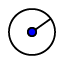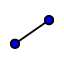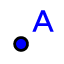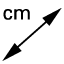# Circle Part 2

Topic:
Circle

## Part II Chords and Perpendicular Radii: Questions 1-6

1. Use(Circle with Center and Radius) to create a 4 unit radius circle. 2.  Use(Segment between Two Points) to create a chord, AB. 3. Use(Perpendicular Bisector) to bisect the chord, click on the two points of the chord. 4.(New Point) Place a point, C, at the bisecting intersection. 5.  Use(Distance or Length) to measure the length of the line segments created by the bisecting line (AC and BC).  Click on the two points to produce a measurement. 6. Repeat this experiment by drawing chord DE and repeating steps 3 to 5.

## Part II Chords and Perpendicular Radii: Question 7

Repeat this experiment by drawing 2 more circles, one smaller and one larger and drawing 2 chords on each circle, and repeat steps 3 to 5.

## Part II Chords and Perpendicular Radii: Question 8

What conclusion can you draw regarding the chord of a circle and the radius that is perpendicular to it?